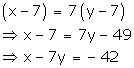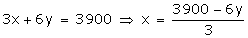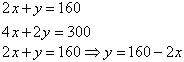# NCERT Solutions for Class 10 Maths Chapter 3 Pair of Linear Equations in Two Variables Ex 3.1

Are you looking for the best Maths NCERT Solutions Chapter 3 Ex 3.1 Class 10? Then, grab them from our page and ace up your preparation for CBSE Class 10 Exams.

NCERT Solutions for Class 10 Maths Chapter 3 Pair of Linear Equations in Two Variables Ex 3.1 are part of . Here are we have given Chapter 3 Pair of Linear Equations in Two Variables Class 10 NCERT Solutions Ex 3.1.

NCERT Solutions for Class 10 Maths Chapter 3 Pair Of Linear Equations In Two Variables are provided here with simple step-by-step explanations. These solutions for Pair Of Linear Equations In Two Variables are extremely popular among class 1 0 students for Math Pair Of Linear Equations In Two Variables Solutions come handy for quickly completing your homework and preparing for exams.

 Board CBSE Textbook NCERT Class Class 10 Subject Maths Chapter Chapter 3 Chapter Name Pair of Linear Equations in Two Variables Exercise Ex 3.1 Number of Questions Solved 3 Category NCERT Solutions

## NCERT Solutions for Class 10 Maths Chapter 3 Pair of Linear Equations in Two Variables Ex 3.1

Page No: 44

Question 1. Aftab tells his daughter, “Seven years ago, I was seven times as old as you were then. Also, three years from now, I shall be three times as old as you will be.” (Isn’t this interesting?) Represent this situation algebraically and graphically.

Solution:
Let the present age of Aftab and his daughter be x and y respectively.
Seven years ago,
Age of Aftab = x – 7
Age of his daughter = y – 7
According to the given condition,Three years hence,
Age of Aftab = x + 3
Age of his daughter = y + 3
According to the given condition,Thus, the given conditions can be algebraically represented as:
x – 7y = -42
x – 3y = 6
x – 7y = -42 ⇒ × = -42 + 7y
Three solutions of this equation can be written in a table as follows:

 x -7 0 7 y 5 6 7

× -3y = 6 ⇒ × = 6 + 3y
Three solutions of this equation can be written in a table as follows:

 x 6 3 0 y 0 -1 -2

The graphical representation is as follows:Concept Insight: In order to represent a given situation mathematically, first see what we need to find out in the problem. Here, Aftab and his daughter’s present age needs to be found so, so the ages will be represented by variables x and y. The problem talks about their ages seven years ago and three years from now. Here, the words ‘seven years ago’ means we have to subtract 7 from their present ages, and ‘three years from now’ or ‘three years hence’ means we have to add 3 to their present ages. Remember in order to represent the algebraic equations graphically the solution set of equations must be taken as whole numbers only for the accuracy. Graph of the two linear equations will be represented by a straight line.

Question 2. The coach of a cricket team buys 3 bats and 6 balls for Rs 3900. Later, she buys another bat and 3 more balls of the same kind for Rs 1300. Represent this situation algebraically and geometrically.

Solution:
Let the cost of a bat and a ball be Rs x and Rs y respectively.
The given conditions can be algebraically represented as:
3x + 6y = 3900
x + 2y = 1300Three solutions of this equation can be written in a table as follows:

 x 300 100 -100 y 500 600 700

x + 2y = 1300 ⇒ x = 1300 – 2y
Three solutions of this equation can be written in a table as follows:

 x 300 100 -100 y 500 600 700

The graphical representation is as follows:Concept Insight:Cost of bats and balls needs to be found so the cost of a ball and bat will be taken as the variables. Applying the conditions of total cost of bats and balls algebraic equations will be obtained. Then, in order to represent the obtained equations graphically take atleast three ordered pairs on both the equations in order to avoid any computational errors.

Question 3. The cost of 2 kg of apples and 1kg of grapes on a day was found to be Rs 160. After a month, the cost of 4 kg of apples and 2 kg of grapes is Rs 300. Represent the situation algebraically and geometrically.

Solution:
Let the cost of 1 kg of apples and 1 kg grapes be Rs. x and Rs. y.
The given condtions can be algebraically represented as:Three solutions of this equation can be written in a table as follows:

 x 50 60 70 y 60 40 20Three solutions of this equation can be written in a table as follows:

 x 70 80 75 y 10 -10 0

The graphical representation is as follows:Concept Insight: cost of apples and grapes needs to be found so the cost of 1 kg apples and 1 kg grapes will be taken as the variables. From the given conditions of collective cost of apples and grapes, a pair of linear equations in two variables will be obtained. Then, in order to represent the obtained equations graphically, take the values of variables as whole numbers only. Since these values are large so take the suitable scale like 1 cm = 20.

We hope the NCERT Solutions for Class 10 Maths Chapter 3 Pair of Linear Equations in Two Variables Ex 3.1 help you. If you have any query regarding NCERT Solutions for Class 10 Maths Chapter 3 Pair of Linear Equations in Two Variables Ex 3.1, drop a comment below and we will get back to you at the earliest.